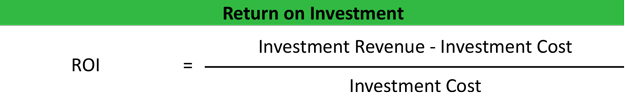# Return on Investment – ROI

Return on investment or ROI is a profitability ratio that calculates the profits of an investment as a percentage of the original cost. In other words, it measures how much money was made on the investment as a percentage of the purchase price. It shows investors how efficiently each dollar invested in a project is at producing a profit. Investors not only use this ratio to measure how well an investment performed, they also use it to compare the performance of different investments of all types and sizes.

For example, an investment in stock can be compared to one in equipment. It doesn’t matter what the type of investment because the return on investment calculation only looks that the profits and the costs associated with the investment.

That being said, the ROI calculation is one of the most common investment ratios because it’s simple and extremely versatile. Managers can use it to compare performance rates on capital equipment purchases while investors can calculate what stock purchases performed better.

## Formula

The return on investment formula is calculated by subtracting the cost from the total income and dividing it by the total cost.As you can see, the ROI formula is very simplistic and broadly defined. What I mean by that is the income and costs are not clearly specified. Total costs and total revenues can mean different things to different individuals. For example, a manager might use the net sales and cost of goods sold as the revenues and expenses in the equation, where as an investor might look more globally at the equation and use gross sales and all expenses incurred to produce or sell the product including operating and non-operating costs.

In this way, the ROI calculation can be very versatile, but it can also be very manipulative depending on what the user wants to measure or show. It’s important to realize that there is no one standardized equation for return on investment. Instead, we’ll look at the basic idea of recognizing profits as a percentage of income. To truly understand the return on an investment presented to you, you have to understand what revenues and costs are being used in the calculation.

## Example

Now that you know that there isn’t a standard equation, let’s look at a basic version without getting into cost and revenue segments. Let’s look at Keith’s Brokerage House for example. Keith is a stockbroker who specializes in penny stocks. Keith made a somewhat risky investment in a liquid metals stock last year when he purchased 5,000 shares at \$1 per share. Today, a year later, the fair market value of per share is \$3.50. Keith sells the share and uses an ROI calculator to measure his performance.As you can see, Keith’s return on investment is 2.5 or 250 percent. This means that Keith made \$2.50 for every dollar that he invested in the liquid metals company. This investment was extremely efficient because it increased 2.5 times.

We can compare Keith’s good choice of liquid metals with his other financial choice of investing in a medical equipment company. He purchased 1,000 shares at \$1 per share and sold them for \$1.25 per share.Keith’s return on this stock purchase was only .25 or 25 percent. It’s still a good return, but nothing compared to the other investment.

## Analysis

Generally, any positive ROI is considered a good return. This means that the total cost of the investment was recouped in addition to some profits left over. A negative return on investment means that the revenues weren’t even enough to cover the total costs. That being said, higher return rates are always better than lower return rates.

Going back to our example about Keith, the first investment yielded an ROI of 250 percent, where as his second investment only yielded 25 percent. The first stock out performed the second one ten fold. Keith would have been better off investing all of his money into the first stock.

The ROI calculation is extremely versatile and can be used for any investment. Managers can use it to measure the return on invested capital. Investors can use it to measure the performance of their stock and individuals can use it to measure their return on assets like their homes.

One thing to remember is that it does not take into consideration the time value of money. For a simple purchase and sale of stock, this fact doesn’t matter all that much, but it does for calculation of a fixed asset like a building or house that appreciates over many years. This is why the original simplistic earnings portion of the formula is usually altered with a present value calculation.

Contents